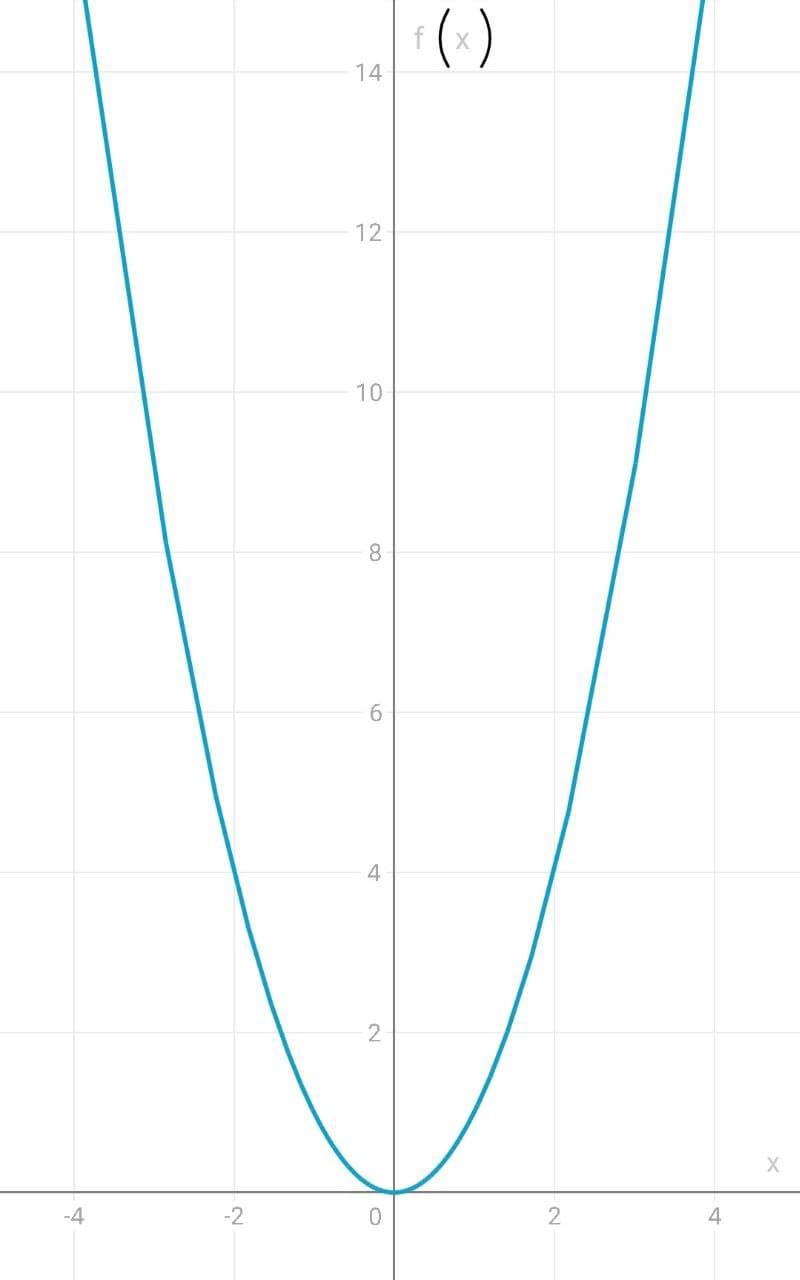dedica66em

2021-12-18

What is the graph of $f\left(x\right)={x}^{2}$?Jeremy Merritt

Expert

The graph of $f\left(x\right)={x}^{2}$ is called a "Parabola." It looks like this:One of the ways to graph this is to use plug in a few x-values and get an idea of the shape. Since the x values keep getting squared, there is an exponential increase on either side of the y-axis. You can see this by plugging in a few values:
When

The same holds true for negative x-values to the left of the y-axis since a negative value squared is positive. For example,Linda Birchfield

Expert

Is there any other graph?nick1337

Expert